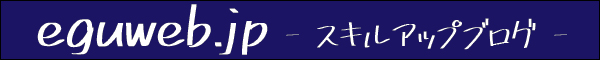## What is ABC Analysis?

“ABC analysis” is the idea that “the top 20% of sales account for 80% of the total”, and is used when formulating strategies based on graphs.

This ABC analysis, also known as ” priority analysis “, is a method of analyzing the financial importance of raw materials, products (merchandise), etc. in inventory management.

It is similar to the chart called ” Pareto analysis “.

## Make an ABC analysis table

First, prepare the table required for ABC analysis. This time, we will download and process the open data “28 Kyoto City Agriculture and Forestry Statistics (Vegetables)”.

## Create “composition ratio” and “cumulative composition ratio”

In order to perform an ABC analysis, we need “composition ratio” and “cumulative composition ratio”, so we will add columns.

This time, we have added columns for “composition ratio” and “cumulative composition ratio”.

## make a composition ratio

Calculate the composition ratio. The ratio of the composition ratio is given by “harvest amount” ÷ “total harvest amount”. Copy the formula to the end with autofill to the cells below. Keep the total cells fixed with absolute references.

When the display format is changed to percentage, the composition ratio (percentage) is displayed.

## Cumulative composition ratio

Calculates the cumulative total of “composition ratio”. The first line is equal to the next composition ratio.

The next line becomes a cumulative total by setting it to “previous composition ratio total + composition ratio”.

After that, if you autofill, it should be “total composition ratio 100%” at the bottom.

## Create a chart for ABC analysis

Among them, we use three items: “item”, “harvest amount”, and “cumulative composition ratio”.

First, in order. First, create a 2-D bar chart using “item” and “yield”.

After selecting Bar Chart, the bar chart is complete.

This time, we will use a “combination chart” instead of this chart.

## Combo chart (grouped column – line on second axis)

Since it is a normal bar graph as it is, change it to a compound graph of “grouped column – line on the second axis”.

“Append another range” to the data range.

Select the Cumulative Percent column.

You now have a combo chart.

## change series

If left as it is, the axis of the harvested amount and the cumulative composition ratio of the graph will have the same value, so we will change it. After selecting “Series”, switch to Cumulative composition ratio.

Change the “axis” part to “right axis”.

Then, the cumulative composition ratio became a line graph on the second axis!

## Completion of the parade diagram

So, the graph of the parade chart of “ABC analysis” is completed!

Based on this graph, what are the top products? What is the cumulative composition ratio of sales? What products make up the top 20%? And so on will be able to analyze!